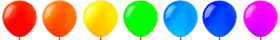# Online math exercises for grade 1Training course. Online math exercises in grade 1. Tasks form in children the foundations of mathematical knowledge and methods of action, the experience of using them to solve educational and practical problems; to realize the potential of students for the formation of the ability to reason logically, to assess the correctness and sufficiency of data for solving educational and practical problems.

The implementation of these tasks is carried out with the help of simulators that will help in the study of such topics as: "Numbers, actions with numbers", "Expressions, equality, inequality".

+ 1

+ 2

+ 3

+ 4

+ 5

+ 6

+ 7

+ 8

+ 9

+ 0

Yes or noOnline assignments in mathematics for the first grade on the topic "Numbers, actions with numbers" form the students' concept of numbers within 100, an understanding of the essence of arithmetic operations of addition and subtraction, the formation of skills in addition and subtraction within 10.

The idea of a natural number, the essence of the arithmetic operations of addition and subtraction and the relationship between these actions in first graders is formed on the basis of visual objects. Considering that a modern child predominantly interacts with two-dimensional ones, online math simulators for the first grade pay more attention to practical exercises with visual objects (geometric bodies, real natural or man-made objects).

## Numerical examples for subtraction

Subtraction
‒ 1

Subtraction
‒ 2

Subtraction
‒ 3

Subtraction
‒ 4

Subtraction
‒ 5

Subtraction
‒ 6

Subtraction
‒ 7

Subtraction
‒ 8

Subtraction
‒ 9

Subtraction
‒ 0In order to prepare for the study of arithmetic operations of addition and subtraction, attention is focused on the formation of calculation techniques within 10 (addition and subtraction of numbers by parts, addition based on the displacement law of addition, subtraction based on the relationship of arithmetic operations of addition and subtraction).

In grade 1, students are introduced to the numbering of numbers within 100; on the basis of practical tasks of the simulator, they comprehend the concept of a discharge, learn to write down binary numbers, master the principle of positional writing of a number. The bit composition of two-digit numbers is the basis for students to compare numbers, add and subtract two-digit numbers in cases based on numbering. If you have cognitive needs and abilities of students, based on the use of visual material, you can perform addition and subtraction of numbers within 100 without going over the digit.

## Calculations

Compare
numbers

Calculations
choose a sign

Verbal counting